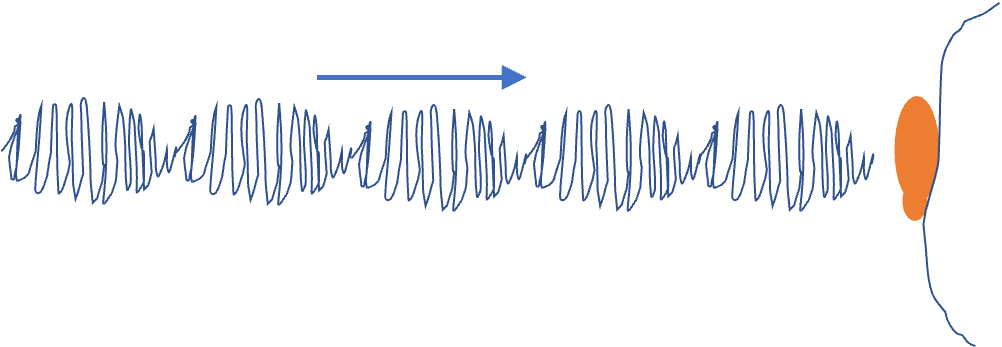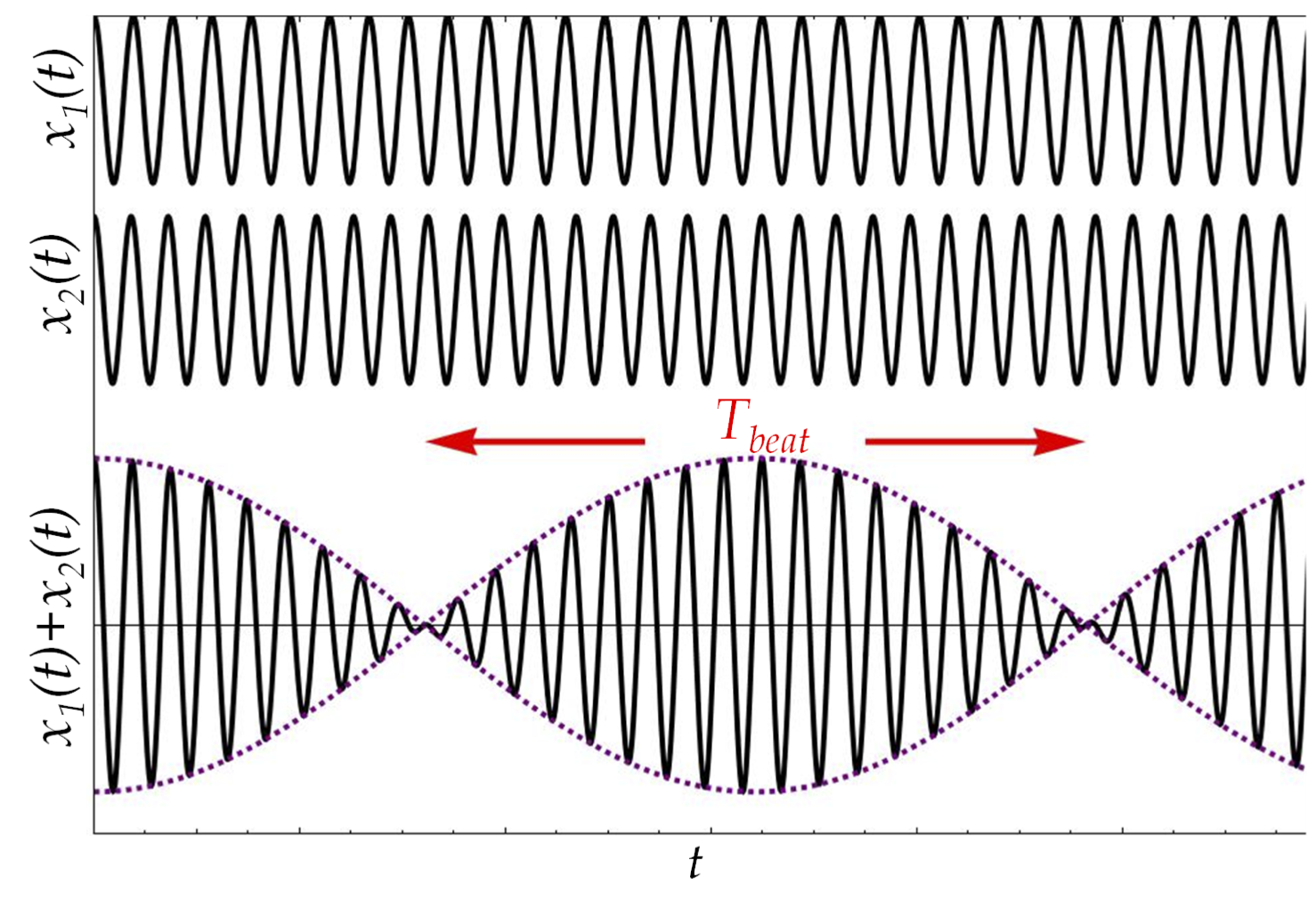## Section14.10Beats

If you simultaneously press two piano keys, you will hear loudness of sound varying with some period. This periodic varying sound is called beats. This is illustrated in Figure 14.10.1. The phenomenon is not restricted to sound waves, but can be observed when two waves of slightly different frequencies strike a detector.Figure 14.10.1. We feel beats in the sound when the loudness of the sound varies periodically with time.

For simplicity, consider two waves $\psi_1$ and $\psi_2$ of different angular frequencies $\omega_1$ and $\omega_2$ and with the same amplitude $A$ meeting at the origin. Suppose, the waves at the origin are causing by the following vibrations.

\begin{align*} \amp \psi_1(0,t) = A\cos(\omega_1 t),\\ \amp \psi_2(0,t) = A\cos(\omega_2 t). \end{align*}

By the superposition principle, the net wave at the origin will be

\begin{equation*} \psi = \psi_1 + \psi_2 = A\left[ \cos(\omega_1 t) + \cos(\omega_2 t) \right]. \end{equation*}

Now, we can use the trigonometric identity

\begin{equation*} \cos A+ \cos B = 2\cos\left(\frac{A-B}{2}\right)\cos\left(\frac{A+B}{2}\right). \end{equation*}

to rewrite the net wave at the origin as

\begin{equation*} \psi = \left\{ 2A\cos\left[ \left( \frac{\omega_1-\omega_2}{2}\right) t\right] \right\} \cos\left[\left(\frac{\omega_1+\omega_2}{2}\right) t\right] \end{equation*}

The result is a net vibration at the average frequency with slowly varying amplitude, the quantity within the curly braces, $\{\}\text{.}$ The amplitude of the wave also varies periodically - we say that the two waves are beating on each other.

Although, two waves of any frequencis can beat with each other, the pronounced beating phenomenon in sound waves we experience in music, happen when two frequencies are close to each other. Therefore, let us write $\omega_1$ and $\omega_2$ as

\begin{align*} \amp \omega_1 = \omega_0 -\frac{\Delta\omega}{2},\\ \amp \omega_2 = \omega_0 +\frac{\Delta\omega}{2}. \end{align*}

Here, $\omega_0$ is their average frequency and $\Delta\omega$ is the difference between the frequencies. Let us write this wave function in the terms of the average frequency $\omega_0$ and the difference $\Delta\omega$ of the frequencies.

\begin{equation*} \psi = \left\{ 2A \cos\left( \frac{\Delta\omega }{2} t\right) \right\} \cos\left( \omega_0 t\right) \end{equation*}

The net vibration consists of fast oscillations at the average frequency $\omega_0$ modulated by a slowly varying cosine as shown in Figure 14.10.2.Figure 14.10.2. The beats as a result of superposition of two vibrations. The plot was made with $f_1$ = 261.63 Hz and $f_2$ = 277.18 Hz, corresponding to $C_4$ and $C_4^\#/D_4^b$ musical notes. The upper graph is the signal at frequency $f_1\text{,}$ the middle at frequency $f_2$ and the bottom graph is the sum of the two. The peaks and troughs of the beats are separated by one beat period as shown.

The amplitude of the fast oscillations is not constant in time but rather varies between $-2A$ and $2A\text{.}$

The largest amplitude oscillations occur at the peak and trough of the slowly varying modulation $\cos\left( \frac{\Delta\omega}{2}t\right)\text{.}$ If the working frequencies are in the audible range, then the peaks and troughs of the slowly varying modulation corresponds to the loudest sound while the sound is faintest when the modulation function is smallest. Thus, sound from two sources of nearby frequencies appear to go loud and soft periodically - the loud sounds are called beats.

Since both the troughs and peaks of $\cos\left( \frac{\Delta\omega}{2}t\right)$ correspond to the loudest sound, there are two beats in one period of this function. Therefore, the beat period is the half of the period of the modulating function $\cos\left( \frac{\Delta\omega}{2}t\right)\text{.}$

\begin{equation*} T_{beat}= \frac{1}{2}\left(\frac{2\pi}{\Delta\omega/2}\right)=\frac{2\pi}{\Delta\omega}. \end{equation*}

Therefore, the beat frequency is the difference in frequencies of the two component vibrations: $\omega_{beat}= \Delta\omega=\left| \omega_1-\omega_2\right|.$ By setting $\omega = 2\pi f\text{,}$ we can write this relation for the beat frequency.

\begin{equation*} \text{Beat frequency: }\ \ f_{\text{beat}}= \left| f_1-f_2\right|. \end{equation*}

The beat phenomenon is very sensitive for detecting the deviation of sound from a pitch. This helps piano tuners as well as members of orchestra to adjust their musical instruments. When an instrument key and a standard reference for that key do not produce any beats, then the two are said to be in tune.

Suppose that a piano key is supposed to be $256\text{ Hz}\text{,}$ but it is out of tune. When a tuning fork of $256\text{ Hz}$ is sounded at the same time as the key, one hears a beat of $2\text{ Hz}$ at a lower pitch than the pitch of the tuning fork.

(a) (i) By how much is the piano key out of tune? (ii) What is the fundamental frequency of the piano string that the key strikes?

(b) Should you tighten or loosen the string to tune in the piano key? Why?

Hint

(a) Use the beat frequency formula. (b) What happens to speed of the wave when tension of the string is increased and how does that impact the fundamental frequency?

(a) $2\text{ Hz}\text{,}$ $254\text{ Hz}\text{,}$ (b) Tighten.

Solution 1 (a)

(a) (i) and (ii): Using the beat frequency formula we find that the frequency of the piano key is off by the beat frequency $2\text{ Hz}\text{.}$ Since the average frequency heard is lower than $256\text{ Hz}\text{,}$ the piano key is actually producing at a lower frequency than $256\text{ Hz}\text{.}$ Therefore, the fundamental frequency of the string corresponding to the key is

\begin{equation*} f = f_\text{tuning fork} - f_\text{beat} = 256-2=254\text{ Hz}. \end{equation*}
Solution 2 (b)

Speed of wave on a string is $v=\sqrt{T/\mu}\text{.}$ Therefore, increasing tension increases speed, and from $f = v/\lambda\text{,}$ that would mean will increase frequency. We want to increase frequency of the string vibration from $254\text{ Hz}$ to $256\text{ Hz}\text{.}$ Therefore, we should tighten the string to tune the piano.

A piano key of $512\text{ Hz}$ is producing 500 Hz sound.

(a) What will be the beat frequency if it is checked against a violin producing $520\text{ Hz}\text{?}$

(b) By what percentage should the tension of the wire be adjusted so that the pitch is adjusted to $512\text{ Hz}\text{?}$ Assume insignificant change in mass per unit length while tightening.

Hint

(a) Use the beat frequency formula. (b) Use $v=\sqrt{T/\mu}$ and $f\lambda=v$ with fixed $\lambda$ and $\mu$ and determine $\Delta T/T$ for corresponding $\Delta f/f\text{.}$

(a) $20\text{ Hz}\text{,}$ (b) $4.8%\text{.}$

Solution 1 (a)

Using the beat frequency formula, we immediately get the answer.

\begin{equation*} f_\text{beat} = 520-500 = 20\text{ Hz}. \end{equation*}
Solution 2 (b)

From $v=\sqrt{T/\mu}$ and $f\lambda=v$ we get

\begin{equation} T = (\mu \lambda^2) f^2.\label{eq-exercise-Using-Beats-to-Tune-a-Piano-tension-frequency}\tag{14.10.1} \end{equation}

Here $\mu \lambda^2$ is fixed. You can use Calculus to get $dT/df\text{,}$ but here I will just say that, let tension be $T+\Delta T$ when frequency is $f+\Delta f\text{.}$ Writing $a$ for the fixed part.

\begin{equation*} T + \Delta T = a (f + \Delta f)^2 = a f^2 + 2 a f \Delta f + a (\Delta f)^2. \end{equation*}

We drop the last term, which will be small compared to the other terms. This gives

\begin{equation*} T + \Delta T \approx a f^2 + 2 a f \Delta f . \end{equation*}

Using Eq. (14.10.1) we get

\begin{equation*} \Delta T = 2 a f \Delta f. \end{equation*}

Divide this by $T$ to get

\begin{equation*} \frac{\Delta T}{ T } = 2 \frac{\Delta f}{f}. \end{equation*}

Now, using the numerical values we obtain

\begin{equation*} \frac{\Delta T}{ T } = 2\times \frac{12}{500} = 0.048 = 4.8%. \end{equation*}